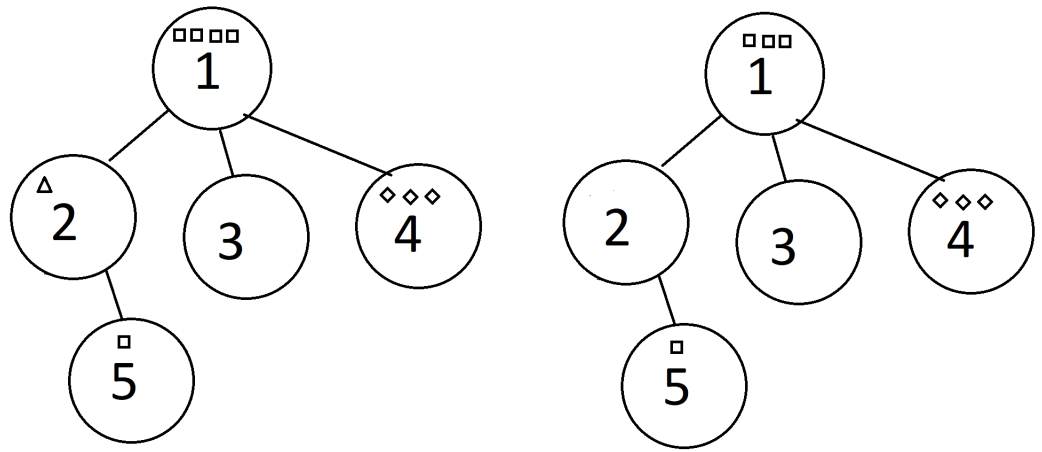시간 제한메모리 제한제출정답맞힌 사람정답 비율
5 초 1024 MB104360.000%

## 문제

Dragonflies can be seen around ponds at Botanic Gardens and Bishan Park. In one of the denser forested areas, Benson the Rabbit has noted down $n$ ponds that the dragonflies fly around. At pond $i$ ($1 ≤ i ≤ n$), there are $b[i]$ bugs that the dragonflies can eat. The bugs at pond $i$ belong to species $s[i]$.

Benson has also noted down $n - 1$ trails. Each trail $j$ ($1 ≤ j < n$) connects 2 distinct ponds $u[j]$ and $v[j]$ bidirectionally. Dragonflies can travel from any pond to any other pond using only the trails.

Benson has captured $d$ dragonflies and intends to release them one at a time at pond $1$. Dragonfly $k$ ($1 ≤ k ≤ d$) has a home pond of $h[k] \ne 1$ and will travel to pond $h[k]$ without visiting any pond more than once using only the trails. These dragonflies will be released in increasing order from dragonfly $1$ to dragonfly $d$. After a dragonfly is released, it will eat a single bug (if there is one or more bugs remaining) at every pond that it visits (including pond $1$), reducing the number of bugs at each of those ponds by $1$ if it is not $0$.

Help Benson determine the number of distinct species of bugs eaten during the journey of each of the $d$ dragonflies.

## 입력

The input format is as follows:

• The first line of input contains $2$ spaced integers $n$ and $d$ respectively.
• The next line of input contains $n$ spaced integers $b, b, \cdots , b[n]$.
• The next line of input contains $n$ spaced integers $s, s, \cdots , s[n]$.
• The next line of input contains $d$ spaced integers $h, h, \cdots , h[d]$.
• The next $n - 1$ lines of input contains $2$ spaced integers each. The $i$th of these lines contains $u[i]$ and $v[i]$ respectively.

## 출력

Output a single line with $d$ spaced integers. The $k$th of these integers should be the number of distinct species of bugs eaten by the $k$th dragonfly.

## 제한

• $2 ≤ n ≤ 2 · 10^5$
• $1 ≤ d ≤ 2 · 10^6$
• $1 ≤ s[i] ≤ n$ (for all $1 ≤ i ≤ n$)
• $0 ≤ b[i] ≤ d$ (for each $1 ≤ i ≤ n$)
• $1 ≤ u[j], v[j] ≤ n$, $u[j] \ne v[j]$ (for each $1 ≤ j ≤ n - 1$)
• $2 ≤ h[k] ≤ n$ (for each $1 ≤ k ≤ n$)

## 서브태스크

번호배점제한
110

$n, d ≤ 1000$

210

$d ≤ 2 · 10^5$, $b[i] = d$ (for all $1 ≤ i ≤ n$)

312

$d ≤ 2 · 10^5$, $b[i] ≤ 10$ (for all $1 ≤ i ≤ n$)

412

$d ≤ 2 · 10^5$, $u[j] = j$, $v[j] = j + 1$ (for all $1 ≤ j ≤ n - 1$)

537

$d ≤ 2 · 10^5$, $s[i] = i$ (for all $1 ≤ i ≤ n$)

616

$d ≤ 2 · 10^5$

73

## 예제 입력 1

5 6
4 1 0 3 1
1 3 2 2 1
2 5 4 3 4 2
5 2
2 1
1 4
1 3


## 예제 출력 1

2 1 2 1 1 0


The input can be visualized using the below figure where □ represents a bug of species 1, △ represents a bug of species 2, and ⋄ represents a bug of species 3.

Initially, the bug configuration matches that of the left diagram. When the first dragonfly flies to pond 2, 1 bug each of species 1 and 2 is eaten. The configuration changes to the right diagram.After that, a dragonfly flies to pond 5. It eats a bug from pond 1 and a bug from pond 5. Note that it does not eat any bugs from pond 2 because there are no more bugs left in pond 2. Although 2 bugs are eaten in total, they are of the same species □, so only 1 distinct species of bugs is eaten.

This testcase is valid for subtasks 1, 3, 6 and 7.

## 예제 입력 2

7 4
0 2 4 4 0 1 3
6 1 6 2 2 2 1
7 5 2 4
4 1
4 5
6 2
1 6
1 3
6 7


## 예제 출력 2

2 1 1 1


This testcase is valid for subtasks 1, 3, 6 and 7.

## 채점 및 기타 정보

• 예제는 채점하지 않는다.
• 이 문제의 채점 우선 순위는 2이다.